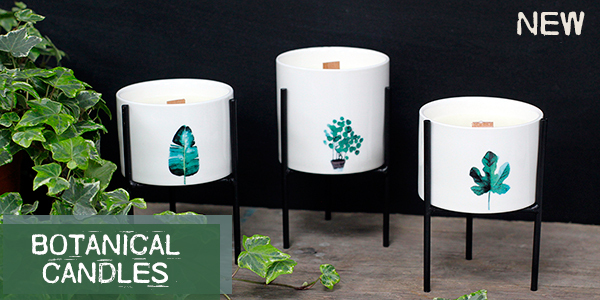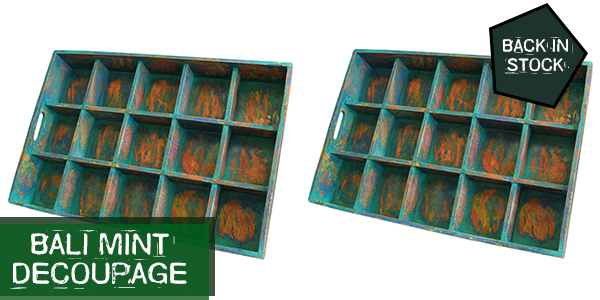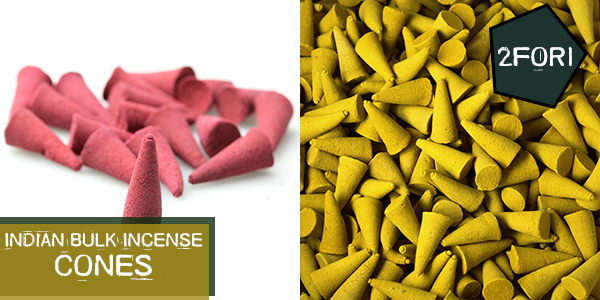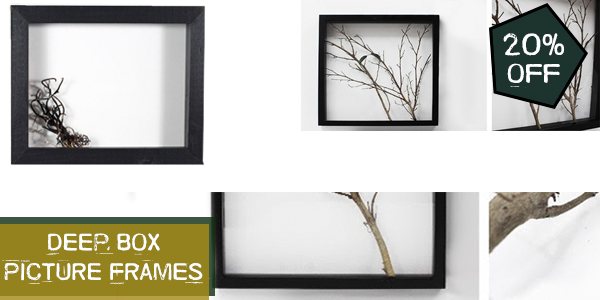Like   Tweet   Pin   +1
 table div table+table+table div table td,table.module-2{width:100%;padding:0}table div table+table+table div table{width:100%;float:none;margin-left:auto;margin-right:auto;padding:0}table div table+table+table div table a{border:0 none;text-decoration:none}table div table+table+table div table img{width:100%!important;border:0 none;text-decoration:none}/* styles */
 /* styles */ Beautifully packed wholesale Soap Flowers are a perfect gift, Valentine's, wedding favours, Mother's day or just a little treat for yourself.
 table div table+table+table+table+table div table td,table.module-4{width:100%;padding:0}table div table+table+table+table+table div table{width:100%;float:none;margin-left:auto;margin-right:auto;padding:0}table div table+table+table+table+table div table a{border:0 none;text-decoration:none}table div table+table+table+table+table div table img{width:100%!important;border:0 none;text-decoration:none}/* styles */
 /* styles */ They are a perfect addition for a relaxing romantic bath. If you sell bathroom products such as soaps and bath bombs these great additions.
 table div table+table+table+table+table+table+table div table td,table.module-6{width:100%;padding:0}table div table+table+table+table+table+table+table div table{width:100%;float:none;margin-left:auto;margin-right:auto;padding:0}table div table+table+table+table+table+table+table div table a{border:0 none;text-decoration:none}table div table+table+table+table+table+table+table div table img{width:100%!important;border:0 none;text-decoration:none}/* styles */
 /* styles */ Set of three fruit box crate made from Albesia wood ethically sourced in Indonesia. These fabulous wooden fruit boxes are perfect for all kinds of storage, from recycling to fruits & vegetables to kindling wood to magazines.
 table div table+table+table+table+table+table+table+table+table div table td,table.module-8{width:100%;padding:0}table div table+table+table+table+table+table+table+table+table div table{width:100%;float:none;margin-left:auto;margin-right:auto;padding:0}table div table+table+table+table+table+table+table+table+table div table a{border:0 none;text-decoration:none}table div table+table+table+table+table+table+table+table+table div table img{width:100%!important;border:0 none;text-decoration:none}/* styles */
 /* styles */ Made in Kathmandu, Nepali with a mixture of Cotton and Pure Hemp, these bags are on trend, fashionable, well designed and... well.. totally awesome
 table div table+table+table+table+table+table+table+table+table+table+table div table td,table.module-10{width:100%;padding:0}table div table+table+table+table+table+table+table+table+table+table+table div table{width:100%;float:none;margin-left:auto;margin-right:auto;padding:0}table div table+table+table+table+table+table+table+table+table+table+table div table a{border:0 none;text-decoration:none}table div table+table+table+table+table+table+table+table+table+table+table div table img{width:100%!important;border:0 none;text-decoration:none}/* styles */
 /* styles */ Luxury Scented Enchanted Glowing Candles are the perfect candle gift. These Candles are unique, the shell is made from a Natural Wax with a combination of Soy Wax and Real Flowers.
 /* styles */ These beautiful and unique Wooden Wick Concrete Candles are one of our favourites. They have a natural and raw appearance that gives a light and loving feel.Botanical Wooden Wick Soy Candles
 /* styles */ We are delighted to present a new range of Wholesale Natural Wax Botanical Candles. Minimalist design pots, double wood wick, full of soybean wax, and fancy iron holder will turn your customers' heads.Bali Mint Decoupage Collection
 /* styles */ Very unique and original Bali Mint Decoupage Collection will make a great addition to any home or gift shop.
 table div table+table+table+table+table+table+table+table+table+table+table+table+table+table+table+table+table+table+table div table td,table.module-18{width:100%;padding:0}table div table+table+table+table+table+table+table+table+table+table+table+table+table+table+table+table+table+table+table div table{width:100%;float:none;margin-left:auto;margin-right:auto;padding:0}table div table+table+table+table+table+table+table+table+table+table+table+table+table+table+table+table+table+table+table div table a{border:0 none;text-decoration:none}table div table+table+table+table+table+table+table+table+table+table+table+table+table+table+table+table+table+table+table div table img{width:100%!important;border:0 none;text-decoration:none}/* styles */
 /* styles */ The spectacular attention to detail gives the wall panel the realism and beauty that you can't get in other wall art. Your customers will be in awe of how much care and talent goes into these wall panels. Each is slightly different, therefore unique.
 table div table+table+table+table+table+table+table+table+table+table+table+table+table+table+table+table+table+table+table+table+table div table td,table.module-20{width:100%;padding:0}table div table+table+table+table+table+table+table+table+table+table+table+table+table+table+table+table+table+table+table+table+table div table{width:100%;float:none;margin-left:auto;margin-right:auto;padding:0}table div table+table+table+table+table+table+table+table+table+table+table+table+table+table+table+table+table+table+table+table+table div table a{border:0 none;text-decoration:none}table div table+table+table+table+table+table+table+table+table+table+table+table+table+table+table+table+table+table+table+table+table div table img{width:100%!important;border:0 none;text-decoration:none}/* styles */
 /* styles */ Our wholesale Indian Wooden Blocks allow you and your customers to design and print your own fabric, papers and other crafty stuff.
 table div table+table+table+table+table+table+table+table+table+table+table+table+table+table+table+table+table+table+table+table+table+table+table div table td,table.module-22{width:100%;padding:0}table div table+table+table+table+table+table+table+table+table+table+table+table+table+table+table+table+table+table+table+table+table+table+table div table{width:100%;float:none;margin-left:auto;margin-right:auto;padding:0}table div table+table+table+table+table+table+table+table+table+table+table+table+table+table+table+table+table+table+table+table+table+table+table div table a{border:0 none;text-decoration:none}table div table+table+table+table+table+table+table+table+table+table+table+table+table+table+table+table+table+table+table+table+table+table+table div table img{width:100%!important;border:0 none;text-decoration:none}/* styles */
 /* styles */ Wedding favour boxes are a lovely way to decorate your reception tables and delight your guests when seated. We have a wonderful range to suit all tastes and wedding themes, so you're certain to find a favour that appeals to you and your customers.Indian Bulk Incense Cones (approx 850 Cones)
 /* styles */ 2 for 1 Wholesale Incense Cones in amazing colours and exotic fragrances. At 25 mm high they are larger than average. Ideal for selling pick and mix style, or repack them and create your own brand. Great value at for approx. 850 pcs ready to retail in a display box.
 table div table+table+table+table+table+table+table+table+table+table+table+table+table+table+table+table+table+table+table+table+table+table+table+table+table+table+table div table td,table.module-26{width:100%;padding:0}table div table+table+table+table+table+table+table+table+table+table+table+table+table+table+table+table+table+table+table+table+table+table+table+table+table+table+table div table{width:100%;float:none;margin-left:auto;margin-right:auto;padding:0}table div table+table+table+table+table+table+table+table+table+table+table+table+table+table+table+table+table+table+table+table+table+table+table+table+table+table+table div table a{border:0 none;text-decoration:none}table div table+table+table+table+table+table+table+table+table+table+table+table+table+table+table+table+table+table+table+table+table+table+table+table+table+table+table div table img{width:100%!important;border:0 none;text-decoration:none}/* styles */
 /* styles */ These Ancient Wisdom 3D Picture Frames definitely have the wow factor. They have a magical effect on the surface to help give the photograph that real 3D effect20% Off Deep Box Picture Frames
 /* styles */ Get your 20% off These wholesale Deep Box Picture Frames are made from sustainable wood and come in either Black or White colours.
 table.module-30{width:99.25%;padding:0}table div table+table+table+table+table+table+table+table+table+table+table+table+table+table+table+table+table+table+table+table+table+table+table+table+table+table+table+table+table+table+table div table{width:99.25%;float:none;margin-left:auto;margin-right:auto;padding:0}table div table+table+table+table+table+table+table+table+table+table+table+table+table+table+table+table+table+table+table+table+table+table+table+table+table+table+table+table+table+table+table div table a{border:0 none;text-decoration:none}table div table+table+table+table+table+table+table+table+table+table+table+table+table+table+table+table+table+table+table+table+table+table+table+table+table+table+table+table+table+table+table div table img{width:100%!important;border:0 none;text-decoration:none}table div table+table+table+table+table+table+table+table+table+table+table+table+table+table+table+table+table+table+table+table+table+table+table+table+table+table+table+table+table+table+table div table td{width:100%;padding:0}/* styles */
 /* styles */ Love makes the world go round. Passion demands romantic gestures, never an easy thing to find. That’s where the gift trade comes in, and if you need something to get your customers besotted hearts beating you’ve come to the right place. At Ancient Wisdom we have heaps of Love & Romance, Candles are Romantic, but you need Hearts and Aroma too.